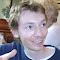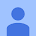James Grime
Mathematician, juggler and comedy nerd - but not necessarily in that order.
Mathematician, juggler and comedy nerd - but not necessarily in that order.
Posts
Post has attachmentPost has attachment
What's so special about 82000?

This is a curious problem, which I saw via   and Thomas Oleron Evans. It's about writing numbers in different bases, and it turns out 82000 is special.

Richard's post is here https://plus.google.com/101584889282878921052/posts/Fni6x2TTeaS and Thomas' original post is here http://www.mathistopheles.co.uk/maths/covering-all-the-bases/solution-covering-all-the-bases/

This is my new favourite thing.﻿Post has attachmentPost has attachmentHey, apparently I’ll be doing one of those Reddit Ask Me Anythings at 9pm GMT (5pm ET). In theory the purpose is to discuss Alan Turing in anticipation of the film, but y'know, whatever. I’ll try and provide a link later.Post has attachment
Gone done a video about Lorenz - the German's "other" cipher machine - even more difficult than Enigma.Post has attachment
This is my new favourite thing. I'm telling everyone about this now. It's the Magic Hexagon - there can be only one.Post has shared content
The arithmetic derivative, the Goldbach conjecture, and the twin prime conjecture

Can you differentiate a number and get a nonzero answer? You can't with the usual kind of differentiation, but you can with the arithmetic derivative.

The basic version of the arithmetic derivative takes positive integers as inputs. The only requirements are the two shown in the picture: (1) the derivative of a prime number is 1, and (2) the product rule holds. It can be proved fairly easily that there is only one function satisfying these requirements. This function is known as the arithmetic derivative, and a formula for it is given at the bottom of the picture in terms of the factorization of n into prime powers. The table shows the values of the derivatives of the first few positive integers; these values will always be other nonnegative integers.

The first person to investigate the arithmetic derivative systematically seems to have been E.J. Barbeau in 1961, although the function had previously appeared in 1950 as a question on a Putnam Prize competition. The first known reference to the function seems to have been in a conference talk in June 1911 by the Spanish mathematician José Mingot Shelly.

The arithmetic derivative has a number of interesting properties, as well as close connections to some famous problems in number theory. It also allows for the study of differential equations involving natural numbers, using the arithmetic derivative in place of the usual derivative. For example, the only solution of the differential equation n'=0 is n=1, and the only solution of n'=1 is for n to be prime. Both of these results are easy exercises.

An excellent introduction to this area is the 2003 paper by Victor Ufnarovski and Bo Åhlander entitled How to Differentiate a Number, which builds on Barbeau's work. The paper contains the above two results but proves many others and offers several conjectures. Some of these conjectures relate to famous open problems in number theory, such as the Goldbach conjecture and the twin prime conjecture.

The Goldbach conjecture is the hypothesis that every even integer greater than 2 can be expressed as the sum of two primes. Ufnarovski and Åhlander conjecture that the (arithmetic) differential equation n'=2b has a positive integer solution for any natural number b > 1; in other words, that any even number greater than 2 shows up as the arithmetic derivative of some natural number. They point out that the Goldbach conjecture implies this conjecture; this is because if 2b is the sum of the primes p and q, then the arithmetic derivative of the number n=pq is 2b. (Note that this is a one-way implication, and it does not mean that a proof of this differential equation identity would prove the Goldbach conjecture.)

The twin prime conjecture is the hypothesis that there are infinitely many primes p such that p+2 is also prime. This is still an open problem, although there have been breakthroughs towards proving this in the last year or so, thanks to work of Yitang Zhang, Terence Tao and others. Ufnarovski and Åhlander conjecture that the differential equation n''=1 has infinitely many solutions, and they point out that the twin prime conjecture would imply this. The reason for this is that if p and p+2 are both primes, then n=2p is a solution to the equation n''=1. (As in the case of the other conjecture, this is a one-way implication.)

There are many generalizations of the arithmetic derivative, some of which are discussed in the paper. For example, the derivative can be extended to negative numbers by changing the sign, and to rational numbers by requiring the derivative to satisfy the quotient rule. It can also be extended to unique factorization domains (UFDs), which are algebraic systems in which elements can be factorized into primes in an essentially unique way. An example of a UFD other than the integers is the Gaussian integers, which is the set of all complex numbers of the form a+bi where a and b are integers.

Wikipedia on the arithmetic derivative: http://en.wikipedia.org/wiki/Arithmetic_derivative

The On-Line Encyclopedia of Integer Sequences on the arithmetic derivative: https://oeis.org/A003415

Ufnarovski and Åhlander's paper: https://cs.uwaterloo.ca/journals/JIS/VOL6/Ufnarovski/ufnarovski.html

A paper by  on a version of the arithmetic derivative for finite fields: https://cs.uwaterloo.ca/journals/JIS/VOL8/Stay/stay44.html

The Goldbach conjecture: http://en.wikipedia.org/wiki/Goldbach_conjecture

The twin prime conjecture: http://en.wikipedia.org/wiki/Twin_primes_conjecture

(Found via Evelyn Lamb on Twitter.)

#mathematics #scienceeverydayPost has shared content
The look-and-say sequence and Conway's Cosmological Theorem

This graphic illustrates the look-and-say sequence, which is a good example of an idea from recreational mathematics that turns out to have hidden depths. To find the next number in the sequence after “111221”, we read out an inventory of the digits in the number (three 1s; two 2s; one 1) and then we represent the inventory itself as the digits of a new number (312211).

The well-known mathematician John H. Conway noticed that, with two exceptions, the long-term behaviour of this iterative process is very interesting, and he proved some nontrivial theorems about it. The two “boring” cases, as Conway calls them, are the number 22, which consists of two 2s and immediately goes into an infinite loop, and the empty string of digits, which never gets anywhere. Any other starting string of digits is defined to be interesting.

Conway's key observation is that after sufficiently many iterations of this procedure, any interesting string of digits will separate into consecutive substrings that never again interact with neighbouring substrings. Using the metaphor of radioactivity, the minimal substrings of this form are known as atoms, and the iterative procedure is known as decay. Because the sequence involves reading previous terms aloud, Conway named this iterative procedure audioactive decay in a 1986 paper. (He enjoys playing with words when introducing new concepts.)

There are 92 basic types of atoms, all of which only involve the digits 1, 2 and 3. These 92 types are known as common elements, and they are nicknamed after the first 92 elements of the periodic table (from hydrogen to uranium). There are also so-called transuranic elements: the Neptunium family (1311222113321132211221121332211n) and the Plutonium family (31221132221222112112322211n), where n can be any digit that is 4 or larger.

Conway's Cosmological Theorem states that every string eventually decays into a compound of common and transuranic elements. (This terminology should not be taken too seriously, and in particular, Conway is not suggesting that the universe is a giant plutonium atom, because that would be ridiculous.) Conway also proves that, starting from an interesting string, the terms eventually grow by about 30 percent on each iteration. More precisely, the length of the string increases asymptotically at each step by a factor of about 1.3035772690, a number known as Conway's constant. This number is an eigenvalue of the 92 by 92 transition matrix associated to the iterative process, and is the unique positive real root of a certain degree 71 polynomial with integer coefficients.

Although it may appear initially that the nature of this problem may depend significantly on the number base chosen, this turns out not to be the case, so long as the base is at least 4. This is because it is provably impossible for any digits other than 1, 2 or 3 to appear in a sequence, unless the initial number contains such a digit or a run of more than three of the same digit. Another remarkable property of audioactive decay, proved by Conway, is that every common element will eventually show up in the decay of any interesting string.

The full statement of the Cosmological Theorem makes a stronger assertion: it states that there exists an integer N such that every string decays in at most N steps to a compound of common and transuranic elements. According to Conway, there used to exist a proof of this fact by him and Richard Parker, as well as another proof by Mike Guy showing that one could take N=24 and that this was the best possible value. Somehow, both these proofs were lost, but fortunately, Shalosh B. Ekhad and Doron Zeilberger showed in their 1997 paper Proof of Conway's lost Cosmological Theorem that N exists and is at most 29. The mathematician Shalosh B. Ekhad (Hebrew for “3B1”) is notable for not being human: it is the name of Zeilberger's computer. Despite this, it has written some single-author papers.

Here's a recent Numberphile video in which Conway talks about this sequence: http://goo.gl/S24CmM. I am grateful to  and  for telling me about the video. I see that the Numberphile channel has just passed the 1 million subscribers mark, so congratulations are in order!

Mathworld on the Cosmological Theorem: http://mathworld.wolfram.com/CosmologicalTheorem.html

Wikipedia on the look-and-say sequence: http://en.wikipedia.org/wiki/Look-and-say_sequence

The look-and-say sequence on the On-Line Encyclopedia of Integer Sequences: http://oeis.org/A005150

Kevin Watkins has some interesting slides and a paper about the Cosmological Theorem on his web page: http://www.cs.cmu.edu/~kw

Ekhad and Zeilberger's paper: http://www.math.rutgers.edu/~zeilberg/mamarim/mamarimhtml/horton.html

#mathematics #scienceeverydayPost has shared content
Secret Project Unveiled

Here is a fun brief video about reflection groups (< 7 min) which I directed and starred in.  I present three examples of reflection groups: dihedral groups, symmetric groups, and hyperoctahedral groups.  The video incorporates all the animations that I created last year which hinted at this secret project.

Many Many Thanks to  who hosted and recorded me, and then professionally assembled all the content with his students Billy Pan and David Hoenig.  Also thanks to the  Center for Teaching and Learning for funding 's work!